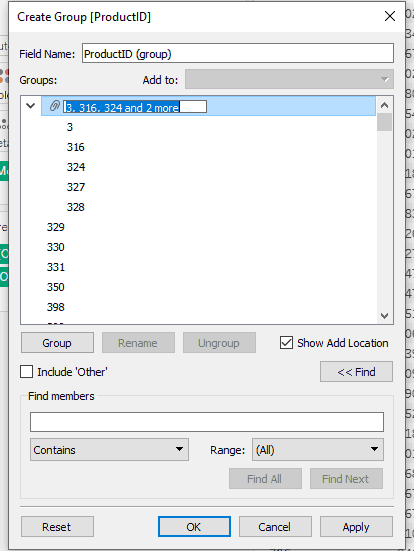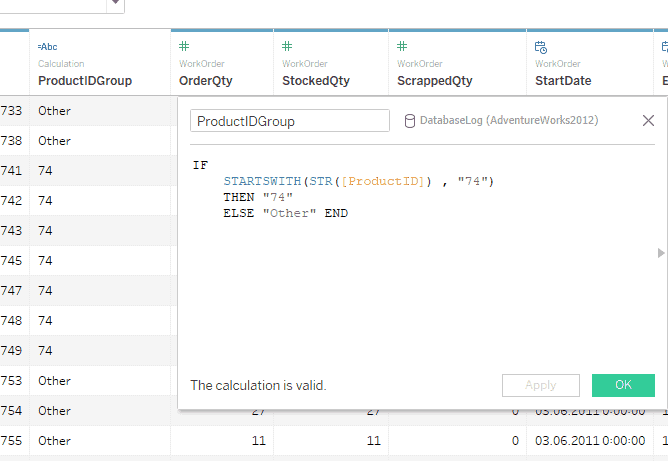# What is the easiest way to make a calculation with a random group of orderid’s in Tableau?

• Tableau FAQs
• December 11, 2018

This post answers how to calculate with a random group of orderid’s in Tableau. This functionality is called grouping in Tableau and can be done in two ways:

Option 1: You can do this using the user interface; for this, you need to follow these steps:

• You need to right-click on the field in which you would like to group
• Then you need to select Create and Group
• in the window that appears, you can select several ProductID values using Ctrl and the left mouse button, and then you will need to click on Group. In the end, you will have a grouping inside the ProductID field.Option 2: The second way is related to writing a calculating field. The result will be about the same but in a different field. Our main task is to define ProductIDs in custom groups; for example, we need to create a group where ProductID starts with “74” and group all the remaining PorudctIDs in the “Other” group.

The logic will be as follows:

``````IF

STARTSWITH(STR([ProductID]) , "74")

THEN "74"

ELSE "Other" END``````

IF  STARTSWITH(STR([ProductID]) , “74”) – Since ProductID is a field in INT format, we need to convert the format of this field to String; for this,[c] we use the STR () function. We need to determine that the string begins with “74”, for this we use STARTWITH (string is a required argument, substring is an essential argument). This expression evaluates to TRUE or FALSE.

THEN “74” – here we indicate what the result will be if the previous expression is TRUE
ELSE “Other” END – and at the end, we need to specify what should happen when the condition evaluates to FALSE. Since we want to define two groups, FALSE will be grouped into the “Other” group.